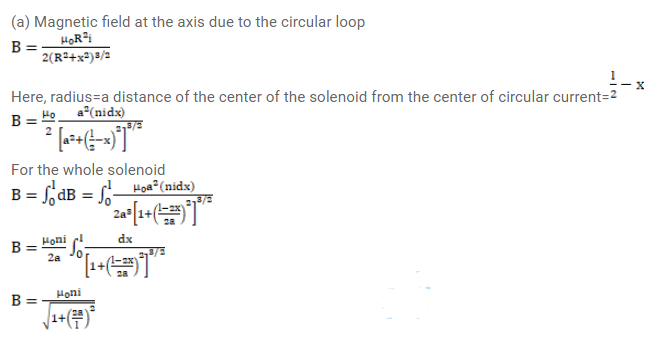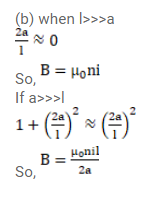# A tightly-wound solenoid of radius a and length I has n turns per unit length.

Question:

A tightly-wound solenoid of radius a and length I has $n$ turns per unit length. It carries an electric current $i$. Consider a length $\mathrm{dx}$ of the solenoid at a distance $x$ from one end. This contains $\mathrm{ndx}$ turns and may be approximated as a circular current i $\mathrm{n} \mathrm{dx}$.

(a) Write the magnetic field at the center of the solenoid due to this circular current. Integrate this expression under proper limits to find the magnetic field at the center of the solenoid.

(b) Verify that if $\mid>>a$, the field tends to Interpret these results.

Solution: